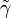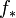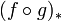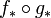# Difference between revisions of "0708-1300/Class notes for Tuesday, January 8"

Announcements go here

## Algebraic Topology

Temporarily, at least, we will no longer assume that all structures are manifolds and that all functions are smooth. However, functions will still be assumed to be continuous.

We will also, temporarily, assume that all spaces are pointed spaces. That is, a space X will be assumed to have a distinguished point x, whether mentioned explicitly or not.

General idea of algebraic topology: to find "functors" from topology to algebra.

Informal definition: A category C consists of

1) a collection Obj(C) of objects

2) a class hom(C) of morphisms between these objects, so that for each X1 and X2 in Obj(C) we get a set mor{X1, X2}

3) Composition laws:

a) a binary function on hom(C): mor(X1, X2) x mor (X2, X3 → mor (X1, X3)

b) Identities (for every X, there is an IX in mor(X,X))

4) Compatibility laws:

a) associativity of composition

b) identities are in fact identities.

"Functors" preserve categories.

Example: Recall that the Brouwer Fixed Point Theorem is implied by the statement that there is no retract from the n-dimensional disc Dn to the (n-1)-dimensional sphere Sn-1. Later, we will prove this last statement in its categorical reformulation: we will find a functor H such that H(D) = {0} and H(Sn-1) = Z. So the existence of a retract would imply that there are some functions f: Z → {0} and g: {0} → Z such that gf is the identity. Clearly this is false, so no retract exists.

Definition: Given (X, x0) (which, by the above comment, we will sometimes write simply as X) we define the "fundamental group" or "Poincaré group" of X:

π1 (X, x0) := { [γ]: γ : [0,1] → X, γ(0) = γ(1) = x0}

Now we have to:

1) Define homotopy and prove that it's an equivalence relation

2) Define a binary operation, show that it's well-defined, and then show that the fundamental group is in fact a group.

3) Demonstrate functoriality

Definition: If f, g : (X, x0) → (Y, y0), we say that "f is homotopic to g" (and we write f ~ g) if there exists a function H : [0,1] × X → Y such that H restricted to {0}×X is f, H restricted to {1}×X is g, and H at any time t is in our category (ie H(t, x0) = y0.)

We know now go through four boring claims, labeled BC1 - BC4. Many have simple visual proofs which I will not reproduce here.

BC1:

~ is an equivalence relation.

Proof of BC1:

a) f ~ f for all functions f. Indeed, set H(t, x) = f(x) for all x.

b) If f ~ g (by a function H) then g ~ f. Indeed, set H'(t,x) = H(1-t, x).

c) If f ~ g (by a function H1) and g ~ h (by a function H2, then f ~ h. Indeed, set H(t, x) = H1(2t,x) if t < ½ and H(t,x) = H2(2t-1, x) if t > ½.

Definition: We define a binary operation on the equivalence classes of paths:

[γ] • [γ'] = [γ • γ']

where the • represents the concatenation of paths. Intuitively, we follow the first path at twice its normal speed, then follow the second, again at twice its normal speed.

BC2: This is well-defined,

BC3: π1 (X, x0) is a group.

Proofette of BC3:

Associativity: Let γ0, γ1 and γ2 be paths. Note that while (γ0 • γ1) • γ2 is not the same path as γ0 • (γ1 • γ2), they are homotopic as paths.

Identity: [e] = [x0], where x0 is the constant path.

Inverse: [γ]-1 = [$\tilde{\gamma}$], where$\tilde{\gamma}$(t) = γ(1-t).

BC4: π1 is a functor.$f_*$ [γ] = [$f_*$ γ] = [f • γ] defines$f_*$ : π1(f) : π1(X) → π1(Y).

To check (all are clear):

1) This is well-defined.

2) It respects compositions.

3) It respects the identity.

4)$(f\circ g)_*$ =$f_*\circ g_*$.

Examples:

1) π1(x0, x0) = {e}.

2) π1 (Rn, 0) = {e}

3) π1 (S1, 1) = Z.